NEET  >  Test: Relative Velocity

# Test: Relative Velocity - NEET

Test Description

## 15 Questions MCQ Test Physics Class 11 - Test: Relative Velocity

Test: Relative Velocity for NEET 2023 is part of Physics Class 11 preparation. The Test: Relative Velocity questions and answers have been prepared according to the NEET exam syllabus.The Test: Relative Velocity MCQs are made for NEET 2023 Exam. Find important definitions, questions, notes, meanings, examples, exercises, MCQs and online tests for Test: Relative Velocity below.
Solutions of Test: Relative Velocity questions in English are available as part of our Physics Class 11 for NEET & Test: Relative Velocity solutions in Hindi for Physics Class 11 course. Download more important topics, notes, lectures and mock test series for NEET Exam by signing up for free. Attempt Test: Relative Velocity | 15 questions in 15 minutes | Mock test for NEET preparation | Free important questions MCQ to study Physics Class 11 for NEET Exam | Download free PDF with solutions
 1 Crore+ students have signed up on EduRev. Have you?
Test: Relative Velocity - Question 1

### A ball A is dropped from a building of height 45 m. Simultaneously another identical ball B is thrown up with a speed 50 m s−1. The relative speed of ball B w.r.t. ball A at any instant of time is (Take g = 10 m s−2).

Detailed Solution for Test: Relative Velocity - Question 1

Here, u= −0 , u= +50ms−1

a= −g , a= −g

uB= uB − u= 50ms−1 − (0)ms−1 = 50ms−1

aB= a− aA = −g − (−g) = 0

∵ vBA = uBA + aBAt(As aBA = 0)

∴ vBA = uBA

As there is no acceleration of ball B w.r.t to ball A, therefore the relative speed of ball B w.r.t ball A at any instant of time remains constant (= 50ms−1).

Test: Relative Velocity - Question 2

### A ball A is thrown up vertically with a speed u and at the same instant another ball B is released from a height h. At time t, the speed of A relative to B is

Detailed Solution for Test: Relative Velocity - Question 2

Taking upwards motion of ball A for time t, its velocity is VA = U - gt.
Taking downwards motion of ball B for time, its velocity is VB = gt.
Relative velocity of A w.r.t. B
=VAB = VA -(-VB) = (u - gt) - (-gt) = u

Test: Relative Velocity - Question 3

### Two cars A and B are running at velocities of 60 km h−1 and 45 km h−1. What is the relative velocity of car A with respect to car B, if both are moving eastward?

Detailed Solution for Test: Relative Velocity - Question 3

Velocity of car A w.r.t. ground

∴ vAG = 60 kmh−1

Velocity of car B w.r.t. ground

∴ vBG = 45 km h−1

Relative velocity of car A w.r.t. B

vAB = vAG + vGB

=vAG − vBG = 15 km h−1 (∵ vGB = −vBG)

Test: Relative Velocity - Question 4

Two cars A and B are running at velocities of 60 km h−1 and 45 km h−1. What is the relative velocity of car A with respect to car B, if car A is moving eastward and car B is moving westward?

Detailed Solution for Test: Relative Velocity - Question 4

Velocity of car A w.r.t. ground

∴ vAG = 60 kmh−1

Velocity of car B w.r.t. ground

∴vBG = -45 km h−1

Relative velocity of car A w.r.t. B

vAB = vAG + vGB

= vAG − vBG = 105 km h−1 (∵ vGB = −vBG)

Test: Relative Velocity - Question 5

On a two-lane road, car A is travelling with a speed of 36 km h-1. Two cars B and C approach car A in opposite directions with a speed of 54 km h-1 each. At a certain instant, when the distance AB is equal to AC, both being 1 km, B decides to overtake A before C does. The minimum required acceleration of car B to avoid an accident is

Detailed Solution for Test: Relative Velocity - Question 5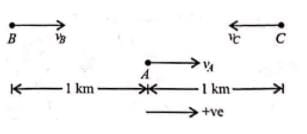Velocity of car A ,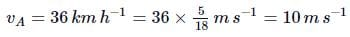Velocity of car B ,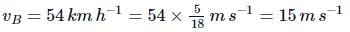Velocity of car C ,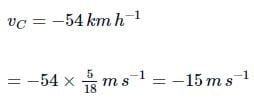Relative velocity of car B w.r.t. car A
vBA = vB−v= 15ms−1−10ms−1 = 5ms−1
Relative velocity of car C w.r.t. car A is
vCA = vC−v= −15ms−1 − 10ms−1=−25ms−1

At a certain instant, both cars B and C are at the same distance from car A

i.e. AB − BC = 1km = 1000m

Time taken by car C to cover 1km to reach car A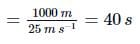In order to avoid an accident, the car B accelerates such that it overtakes car A in less than 40s. Let the minimum required acceleration be a. Then,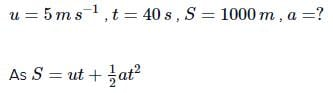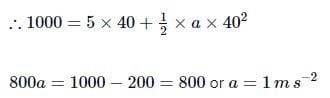Test: Relative Velocity - Question 6

A bird is tossing (flying to and fro) between two cars moving towards each other on a straight road. One car has speed of 27 km h−1 while the other has the speed of 18 km h−1. The bird starts moving from first car towards the other and is moving with the speed of 36 km h−1 when the two were separated by 36 km. The total distance covered by the bird is

Detailed Solution for Test: Relative Velocity - Question 6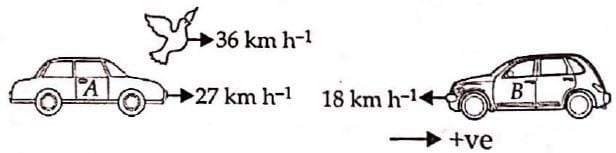Velocity of car A, v= +27kmh−1

Velocity of car B, v= −18kmh−1

Relative velocity of car A with respect to car B

=v− vB = + 27kmh−1  (−18kmh−1) = 45kmh−1
Time taken by the two cars to meet = 36 km/45 km h-1  = 0.8

Thus, distance covered by the bird
= 36kmh−1 x 0.8h = 28.8km

Test: Relative Velocity - Question 7

A police van moving on a highway with a speed of 30km h−1 fires a bullet at a thief's car speeding away in the same direction with a speed of 192km h−1. If the muzzle speed of the bullet is 150ms−1 , with what relative speed does the bullet hit the thief's car?

Detailed Solution for Test: Relative Velocity - Question 7

Speed of police van w.r.t. ground

∴ vPG = 30kmh−1

Speed of thief’s car w.r.t. ground

∴ vTG = 192kmh−1

Speed of bullet w.r.t. police van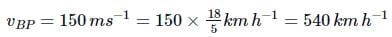Speed with which the bullet will hit the thief’s car will be

vBT = vBG + vGT = vBP + vPG + vGT

= 540kmh−1 + 30kmh−1 − 192kmh−1

(∵ vGT = −vTG)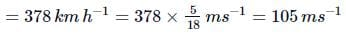Test: Relative Velocity - Question 8

A bus is moving with a speed of 10ms−1 on a straight road. A scooterist wishes to overtake the bus in 100s. If the bus is at a distance of 1km from the scooterist with what speed should the scooterist chase the bus?

Detailed Solution for Test: Relative Velocity - Question 8

Let vs be the velocity of the scooter, the distance between the scooter and the bus = 1000m,

The velocity of the bus = 10ms−1

Time taken to overtake = 100s

Relative velocity of the scooter with respect to the bus = (v− 10)

1000/(vs − 10) = 100s

= vs = 20ms−1

Test: Relative Velocity - Question 9

Two towns A and B are connected by a regular bus service with a bus leaving in either direction every T minutes. A man cycling with a speed of 20 kmh-1 in the direction A to B notices that a bus goes past him every 18 min in the direction of his motion, and every 6 min in the opposite direction. The period T of the bus service is

Detailed Solution for Test: Relative Velocity - Question 9

Let vkmh−1 be the constant speed with which the bus travel ply between the towns A and B .

Relative velocity of the bus from A to B with respect to the cyclist = (v − 20)kmh−1

Relative velocity of the bus from B to A with respect to the cyclist = (v + 20)kmh−1

Distance travelled by the bus in time T (minutes) = vT

As per question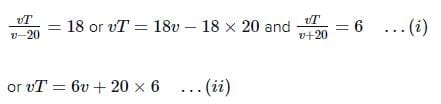Equating (i) and (ii) , we get

= 18v − 18 x 20 = 6v + 20 × 6

or 12v = 20 x 6 + 18 x 20 = 480 or v = 40 kmh−1

Putting this value of v in (i) , we get

40T = 18 x 40 − 18 x 20 = 18 x 20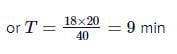Test: Relative Velocity - Question 10

A 175m long train is travelling along a straight track with a velocity 72km−1h. A bird is flying parallel to the train in the opposite direction with a velocity 18km−1h. The time taken by the bird to cross the train is

Detailed Solution for Test: Relative Velocity - Question 10

Velocity of train,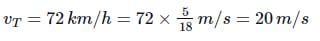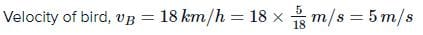Since bird is flying parallel to train in opposite direction.

∴ Relative velocity of bird w.r.t. train = vB + vT = 25m/s Train’s length = 175m

Time taken by the bird to cross the train is = 175/25 = 7s

Test: Relative Velocity - Question 11

Two parallel rail tracks run north-south. On one track train A moves north with a speed of 54kmh−1 and on the other track train B moves south with a speed of 90kmh−1. The velocity of train A with respect to train B is

Detailed Solution for Test: Relative Velocity - Question 11

Let the positive direction of motion be from south to north. Velocity of train A with respect to ground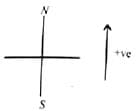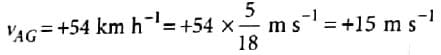Velocity of train B with respect to ground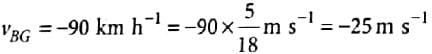Relative velocity of train A with respect to train B is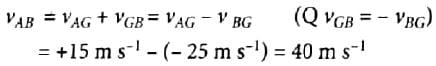Test: Relative Velocity - Question 12

Two parallel rail tracks run north-south. On one track train A moves north with a speed of 54 kmh−1 and on the other track train B moves south with a speed of 90kmh−1.  What is the velocity of a monkey running on the roof of the train A against its motion with a velocity of 18 kmh-1 with respect to the train A as observed by a man standing on the ground?

Detailed Solution for Test: Relative Velocity - Question 12

Let the velocity of the monkey with respect to ground be vMG
Relative velocity of the monkey with respect to the train A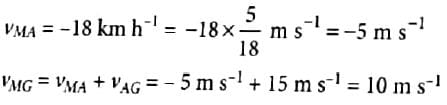Test: Relative Velocity - Question 13

A train A which is 120m long is running with velocity 20m/s while train B which is 130m long is running in opposite direction with velocity 30m/s. What is the time taken by train B to cross the train A?

Detailed Solution for Test: Relative Velocity - Question 13

Length of train A = 120m
Velocity of train A, v= 20m/s
Length of train B = 130m
Velocity of train B, v= 30m/s
Relative velocity of B w.r.t. A = v+ v= 50m/s
(trains move in opposite directions)
Total path length to be covered by B = 130 + 120 = 250m

∴ Time taken by train B = 250 m/50m/s = 5 s

Test: Relative Velocity - Question 14

A jet airplane travelling at the speed of 500kmh−1 ejects its products of combustion at the speed of 1500kmh−1 relative to the jet plane. The speed of the products of combustion with respect to an observer on the ground is

Detailed Solution for Test: Relative Velocity - Question 14

Veloity of jet plane w.r.t ground vjG = 500 km h-1
Velocity of products of combustion w.r.t jet plane vCJ = -1500 kmh-1
∴ Velocity of products of combustion w.r.t ground is vCG = vCJ + vJG = - 1500kmh-1 + 500 kmh-1
= -1000 km h-1
-ve sign shows that the direction of products of combustion is opposite to that of the plane
∴ Speed of the products of combustion w.r.t ground = 1000 km h-1

Test: Relative Velocity - Question 15

On a long horizontally moving belt, a child runs to and fro with a speed 9kmh−1 (with respect to the belt) between his father and mother located 50m apart on the moving belt. The belt moves with a speed of 4kmh−1. For an observer on a stationary platform, the speed of the child running in the direction of motion of the belt is

Detailed Solution for Test: Relative Velocity - Question 15

Figure shows conditions of the question.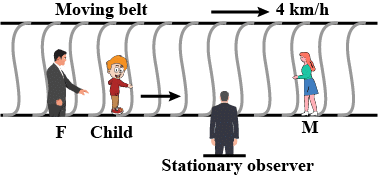In this case,

Speed of belt w.r.t. ground

∴ vBG = 4kmh−1

Speed of child w.r.t. belt

∴ vCB = 9kmh−1

∴ For an observer on a stationary platform, speed of child running in the direction of motion of the belt is

vCG = vCB + vBG = 9kmh−1 + 4kmh−1

= 13kmh−1

## Physics Class 11

130 videos|483 docs|210 tests
Information about Test: Relative Velocity Page
In this test you can find the Exam questions for Test: Relative Velocity solved & explained in the simplest way possible. Besides giving Questions and answers for Test: Relative Velocity, EduRev gives you an ample number of Online tests for practice

## Physics Class 11

130 videos|483 docs|210 tests

### How to Prepare for NEET

Read our guide to prepare for NEET which is created by Toppers & the best Teachers(Scan QR code)